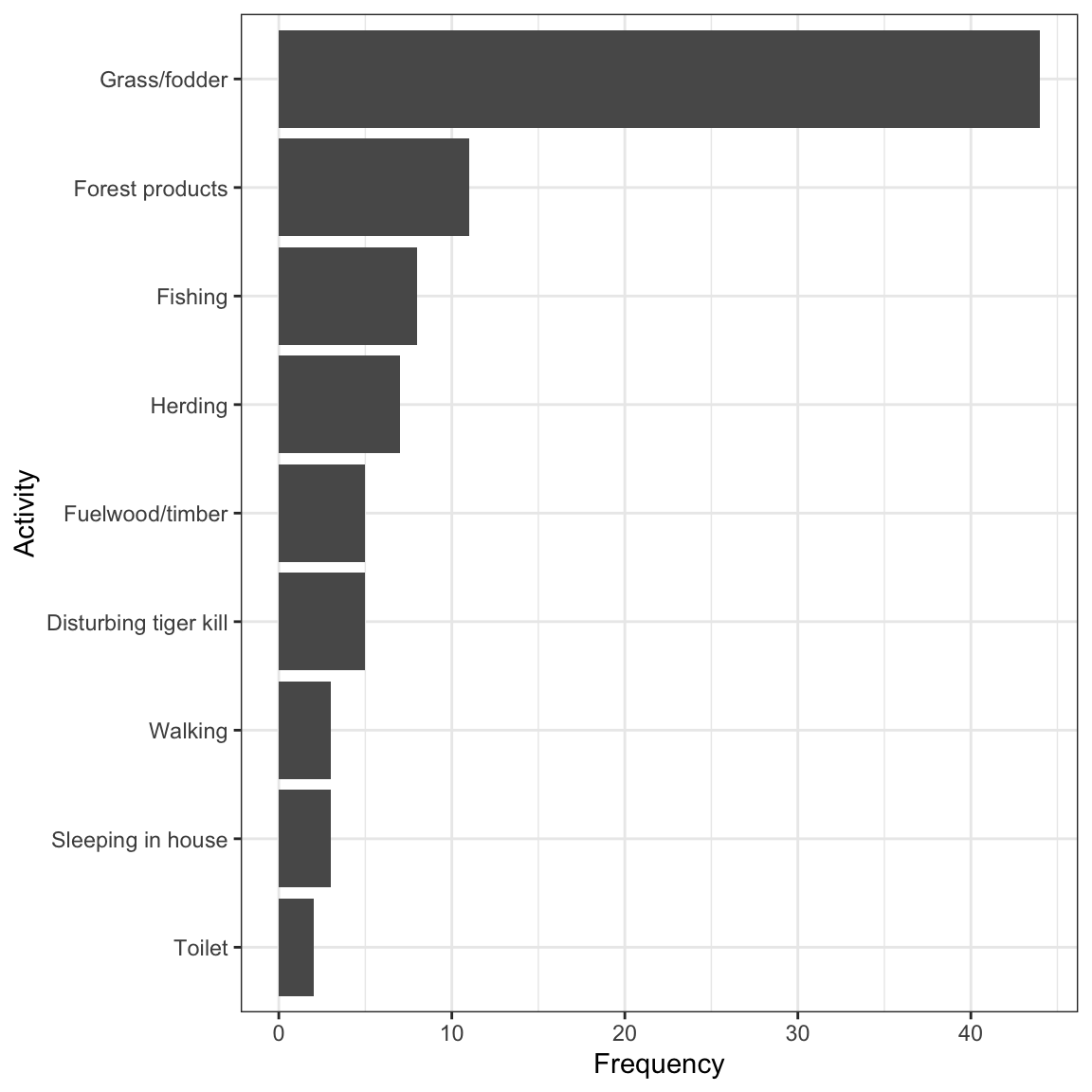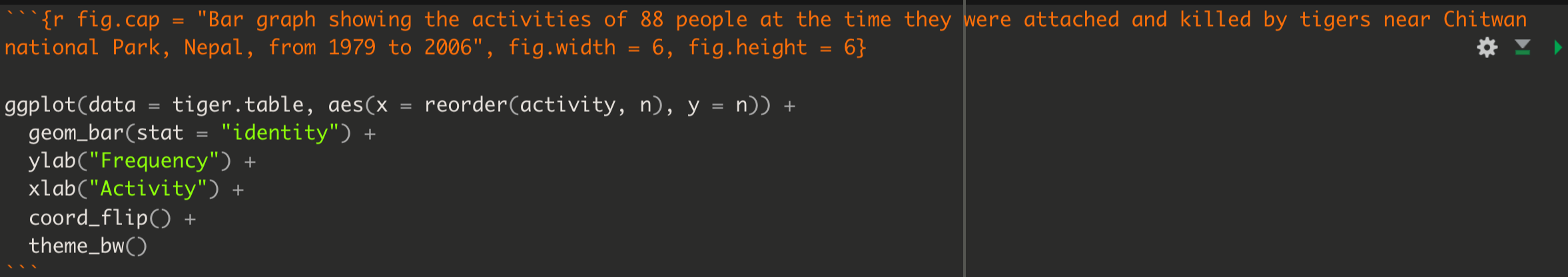## 6.4 Create a bar graph

We use a bar graph to visualize the frequency distribution for a single categorical variable.

We’ll use the ggplot approach with its geom_bar function to create a bar graph. The ggplot function comes with the ggplot2 package, which itself is loaded as part of the tidyverse.

To produce the bar graph, we use a frequency table as the input. Thus, let’s repeat the creation of the “tiger.table” from the preceding section, but this time we exclude the adorn_totals line of code, because we don’t want the “total” row to be plotted in the bar graph.

tiger.table <- tigerdeaths %>%
count(activity, sort = TRUE) %>%
mutate(relative_frequency = n / sum(n))

Recall that the “tiger.table” is a sort of summary presentation of the “activity” variable:

tiger.table
## # A tibble: 9 × 3
##   activity                  n relative_frequency
##   <chr>                 <int>              <dbl>
## 1 Grass/fodder             44            0.5
## 2 Forest products          11            0.125
## 3 Fishing                   8            0.09091
## 4 Herding                   7            0.07955
## 5 Disturbing tiger kill     5            0.05682
## 6 Fuelwood/timber           5            0.05682
## 7 Sleeping in house         3            0.03409
## 8 Walking                   3            0.03409
## 9 Toilet                    2            0.02273

It shows the total counts (frequencies) of individuals in each of the nine “activity” categories.

And although in the code chunk below you’ll see that we provide an “x” and a “y” variable for creating the graph, remember that we’re really only visualizing a single categorical variable.

Let’s provide the code first, and explain after.

ggplot(data = tiger.table, aes(x = reorder(activity, n), y = n)) +
geom_bar(stat = "identity") +
ylab("Frequency") +
xlab("Activity") +
coord_flip() +
theme_bw()Figure 6.1: Bar graph showing the activities of 88 people at the time they were attached and killed by tigers near Chitwan national Park, Nepal, from 1979 to 2006

All figures produced using the ggplot2 package start with the ggplot function. Then the following arguments:

• The tibble (or dataframe) that holds the data (“data = tiger.table”)
• An “aes” argument (which stands for “aesthetics”), within which one specifies the variables to be plotted; here we’re plotting the frequencies from the “n” variable in the frequency table as the “y” variable, and the “activity” categorical variable as the “x” variable. To ensure the proper sorting of the bars, we use the reorder function, telling R to reorder the activity categories according to the frequencies in the n variable
• Then there’s a plus sign (“+”) to tell the ggplot function we’re not done yet with our graph - there are more lines of code coming (think of it as ggplot’s version of the “pipe”)
• Then the type of graph, which uses a function starting with “geom”; here we want a bar graph, hence geom_bar
• The geom_bar function has its own argument: “stat = ‘identity’” tells it just to make the height of the bars equal to the values provided in the “y” variable, here n.
• The ylab function sets the y-axis label
• The xlab function sets the x-axis label
• The coord_flip function tells it to rotate the graph horizontally; this makes it easier to fit the activity labels on the graph
• Then the theme_bw function indicates we want a simple black-and-white theme

There you have it: a nicely formatted bar graph!

REMINDER Don’t forget to include a good figure caption! Here’s a snapshot of the full code chunk that produced the bar graph above:Figure 6.2: Example code chunk for producing a good bar graph

1. Bar graph: Try creating a bar graph using the birds dataset, which includes data about four types of birds observed at a wetland.